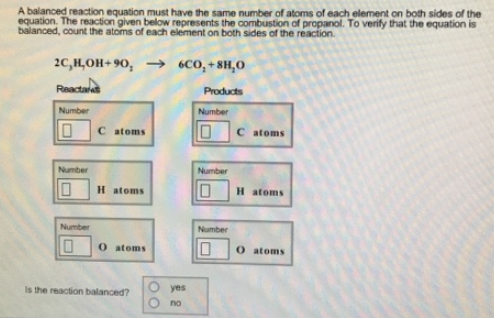# Problem: A balanced reaction equation must have the same number of atoms of each element on both sides of the equation. The reaction given below represents the combustion of propanol. To verify that the equation is balanced, count the atoms of each element on both sties of the reaction. 2C3H7OH + 9O2 → 6CO2 + 8H2O Is the reaction balanced?              • yes               • no

###### FREE Expert Solution
91% (204 ratings)###### Problem Details

A balanced reaction equation must have the same number of atoms of each element on both sides of the equation. The reaction given below represents the combustion of propanol. To verify that the equation is balanced, count the atoms of each element on both sties of the reaction.

2C3H7OH + 9O2 → 6CO2 + 8H2O

Is the reaction balanced?

• yes

• no